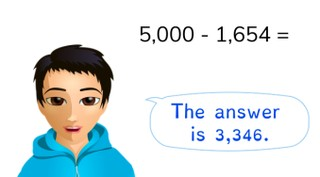Subtracting from a thousands number

# Subtracting from a thousands number

Students learn to subtract from a thousands number.

No account needed.8,000 schools use Gynzy92,000 teachers use Gynzy1,600,000 students use Gynzy

## General

The students learn to subtract from a thousands number.

## Standards

CCSS.Math.Content.4.NBT.B.4

## Learning objective

Students will be able to subtract to 10,000 from thousands numbers.

## Introduction

Have the students solve the story problem.

## Instruction

Explain that to solve the problem 8,000 - 1,949 you first subtract the thousands numbers, then the hundreds numbers, tens numbers, and ones values. Then discuss the problem 2,000 - 1,090 together. Show how the children solve the problem and ask the students how they would solve it. Next, have the students solve the next few problems on their own. Explain that for a story problem you must first determine what type of problem it is. Then you see what numbers you need from the story, and you state the problem. The last step is solving the problem. You can do this for example by first doing 2,000 - 1,000 and then 1,000 - 100. Discuss the problem with the animal images together and have the students solve the next problem on their own.

Check whether the students can subtract from a thousands number by asking the following question:
- Which method do you use to solve this problem in steps? (3,000 - 1,011 = )

## Quiz

The students are given ten questions in which they practice with visual, abstract, and story problems.

## Closing

Discuss again the important of being able to subtract from a thousands number. As a closing activity, have the students solve the story problem in pairs.

## Teaching tips

Have students that have difficulty with subtracting from a thousands number first practice subtracting to 1,000 from a hundreds number.

### The online teaching platform for interactive whiteboards and displays in schools

• Save time building lessons

• Manage the classroom more efficiently

• Increase student engagement